## Journal of Computer Engineering & Information TechnologyISSN : 2324-9307

All submissions of the EM system will be redirected to Online Manuscript Submission System. Authors are requested to submit articles directly to Online Manuscript Submission System of respective journal.

Research Article, J Comput Eng Inf Technol Vol: 5 Issue: 2

# Synchronizing Two Frequently-Cited High-Capacity Image Hiding Methods (Modulus-Based Vs. LSB-Based)

 Ja-Chen Lin* Professor, Department of Computer and Information Science, National Chiao Tung University, Hsinchu, Taiwan 300, Taiwan Corresponding author : Ja-Chen Lin Professor, Department of Computer and Information Science,National Chiao Tung University, Hsinchu, Taiwan 300,Taiwan Fax: +886-3-5721490 E-mail: jclin@cis.nctu.edu.tw Received: December 31, 2015 Accepted: April 05, 2016 Published: April 11,2016 Citation: Lin JC (2016) Synchronizing Two Frequently-Cited High-Capacity Image Hiding Methods (Modulus-Based Vs. LSB-Based). J Comput Eng Inf Technol 5:2. doi:10.4172/2324-9307.1000145

## Abstract

Synchronizing Two Frequently-Cited High-Capacity Image Hiding Methods (Modulus-Based Vs. LSB-Based)

This study briefly reviews and then connects a Modulus-based image hiding method published in 2003 and an LSB-based (Least- Significant-Bits) image hiding method published in 2004. Both methods are frequently cited; and the reason is because of their high hiding capacity (the size of the data can be hidden in an image) and low distortion to the host image; along with the benefit of being simple. This study synchronizes the two methods as one simple method so that the PSNR prediction formula of the 2003 method can also be used by the 2004 method to predict the PSNR of the stego image.

### Keywords: Data hiding; Least Significant Bits (LSB) based; Modulus based; PSNR estimation formula; Mean Absolute Error (MAE); Estimation formula

#### Keywords

Data hiding; Least Significant Bits (LSB) based; Modulus based; PSNR estimation formula; Mean Absolute Error (MAE); Estimation formula

#### Introduction

Data hiding has been a popular research topic for decades [1- 10]. In data hiding, people try to hide secret data in some cover media. High hiding capacity and low distortion to the cover media are usually required when a method is designed. Both the Modulusbased hiding method  and the LSB-based (Least-Significant-Bits) hiding method  are of high hiding capacity and low distortion; so these two methods are frequently cited. The study here synchronizes these two methods as one simple method; therefore, the users of the LSB method  can also use all mathematical formulas listed ;especially, the PSNR-prediction formula . Moreover, we will also design here a new formula which is useful in predicting the Mean Absolute Error (MAE) of the stego images. Of course, this new formula can also be applied to both methods [1,2].
Notably, so far, most of the research papers in image hiding field show PSNR values (computed from Mean Square Error (MSE) values), rather than MAE values, of the stego images. Willmott and Matsuura  suggest the use of MAE to replace MSE or square root of MSE (the RMSE); whereas some  still suggests the favor of RMSE. In certain applications or in other fields, some researchers begin to provide MAE values or list both MAE and PSNR simultaneously in the same paper; for example, the audio hiding system , or the computer-aided breast tumor segmentation system . Therefore, we try to list both MAE and PSNR in our report here so that, in the future, the researchers can use these MAE and PSNR values to compare against their own methods’ MAE and PSNR.
The PSNR-bpp performance of some other high-capacity and low-distortion methods [4-8] recently appeared in literature are also compared against that of the simple methods [1,2]. The methods being compared include the multi-layer embedding method of Tang, Hu et al. ; the tunable method of Kanan and Nazeri  who use genetic algorithm to get nearly optimal search; the adaptive method designed by Maleki et al. , together with the other adaptive method of Sadashiv and Rao . We will show that the simple methods [1,2] are still very competitive nowadays, although they were originally designed in 2003-2004.

#### Materials and Methods

Summarizing Thien and Lin’s Module-based hiding method
Consider hiding a sequence of digits of base-m (each digit is in the range {0,1,2,3,4,5,…,m−2, m−1}); then embed each digit x in a pixel y of a given host image (one digit per pixel). Due to hiding, original pixel value y is changed to the stego pixel value y′. It is easy to prove that the embedding formula used by Thien and Lin  can be simplified as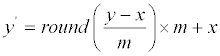(1)
where the round operator rounds its argument to the nearest integer. The extraction of the hidden digit x from stego pixel y′ is also very simple, just use(2)
Remark 1: Of course, if the new pixel value y′ is less than 0 (or larger than 255), do the adjustment by using y ′+m (or y ′−m) as the final pixel value. Notably, this ±m adjustment will not affect the value x extracted by (2) due to modm operation.
A particularly important quantity property provided by Thien and Lin  is:
Property 3 of Thien and Lin’s Module-based hiding method: If the embedded data {x} is uniformly distributed, then after hiding in each pixel a message digit, the expected PSNR of the whole stego image is approximately
PSNR=10Log{12(2552)/(m2− 1)} when m is odd; (3)
PSNR=10Log{12(2552)/(m2+2)} when m is even. (4)
End of Property 3 of Thien and Lin’s Module-based hiding method: Notably, by Equation (4), the PSNR of the stego image is
10Log{12(2552)/(m2+2)}= 51.1411; 46.3699; 40.7272; and 34.8064 (5)
for hiding 1 bit per pixel (bpp), hiding 2 bpp, hiding 3 bpp, and hiding 4 bpp, respectively; because we only need to plug in Eq. (4) the parameter value
m=2k= 2, 4, 8, 16, (6)
respectively. These PSNR values are extremely close to the experimental values obtained in Tables 1 and 2 of Chan CK et al.’s research , the experimental values they obtained were {51.1410, 46.3699, 40.7271, 34.8062} for host image Lena, {51.1414, 46.3691, 40.7253, 34.8021} for host image Baboon, {51.1405, 46.3700, 40.7273, 34.8065} for host image Jet, {51.1410, 46.3702, 40.7270, 34.8060} for image Scene.)Table 1: The PSNR-bpp performance of the method here vs. the method .Table 2: MAE (Mean Absolute Error) comparison.
Summarizing LSB-based hiding method
Motivated by their own graceful observation , Chan and Cheng  proposed a method in which each host pixel hides a k-bits binary-value x. The method can also be summarized as Eq. (1) and (2); except that only uses
m=2k ∈{2,4,8,16}.
Below we explain why the hiding method in  can also be described as Eq. (1) and (2), using m=2k. Notably, Eq. (5) of  also uses the same extraction operation x=(y′)modm = ( y′)mod 2k stated here in Eq. (2) to extract data digit x. So, we only need to explain why the stego pixel value y′  is identical to the one produced here by Eq. (1). Since also uses x=(y′)modm to extract x, the stego pixel value y′ of must satisfy y=lm+x for some integer l. In Chan CK, Cheng LM research , their design to choose the integer l is according to the merit that the distance |y′-y| between the stego pixel value y′=lm+x and the original pixel value y should be as small as possible. The rounding function in Eq. (1) above chooses l asand this l can achieve this minimal distortion goal. To see the reason, letso the original pixel value y is y=nm+a for some nonnegative integer. Here,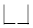is the round-down operator, also known as the floor function. The distortion caused by embedding is
|y′-y| =|(l-n)m+(x-a)|.
Traditional LSB method just let l=n, i.e. the integer portion of (y/m); and this implies |y′-y| =|(x-a)| which is at most m-1 because both x and a are in {0,1,....,m-1}. Chan and Cheng  choose l by considering not only n, but also other integers near n, i.e., n+1 and n-1. Since more possible candidates are considered for l, the resulting system improves the distortion of traditional LSB. As for Eq. (1) above, since
x ∈{0,1,…,m-1} and a ∈ {0,1,…,m-1} ⇒ –m<(a-x)<m,
we see that round((a-x)/m) is either -1 or 0 or 1. Hence, the y′ of Eq. (1) isi.e, the y′ of Eq. (1) is also an lm+x with possible values of l being n-1 or n or n+1. Finally, the rounding operator automatically adjusts the value of l to obtain minimal distortion between y and y′. For example, if a−x ≥ 0.5m, then round ((a-x)/m)=round(0.5…)=1, then Eq. (1) becomes y′=(n+1)m+x, so y′−y= ((n+1)m+x) −(nm+a) =m−(a−x)=m−(m*(0.5…)) becomes a number between 0 and 0.5m. The distortion is thus |y′-y| ≤ 0.5m. This is better than the distortion |(nm+x)−y|=|(nm+x) −(nm+a)|=|x−a|≥ 0.5m caused by using l=n, and also better than the distortion |((n−1))m+x)−y|=|−m+x− a|=|m+a−x|≥ 1.5m caused by using l=n-1. Therefore, for the case a−x ≥ 0.5m, the y′ created by Eq. (1) is identical to the best lm+x chosen by the method . The case a−x≤−0.5m can be proved likewise to get minimal distortion by using l=n−1 which is also automatically determined by the rounding operator of Eq. (1). Finally, analogous prove also shows that the case −0.5m <a−x<−0.5m will get minimal distortion by using l=n which is also automatically determined by the rounding operator of Eq. (1). The tedious proof of these two cases is omitted to save paper length. Interested readers can see the proof of the Lemma later to get more idea of how to prove here for the case a−x ≤−0.5m or the case −0.5m <a−x<−0.5m.
As a final remark, Eq. (11) stated that the worst-case MSE of their method is a constant q times the worst-case MSE of the traditional LSB method , with q being 1, 4/9, 16/49, and 64/225; when each pixel hides 1, 2, 3, and 4 bits; respectively.
Comparing methods (Modulus-based vs. LSB-based)
The two methods proposed by Thein and Lin and Chan and Chang were designed individually [1,2]; and both are submitted in 2002, the work of Chan and Cheng  was submitted 80 days earlier, although the study by Thein and Lin  was published earlier due to the direct acceptance. Both Thein and Lin  provide some mathematical properties emphasizing the estimated PSNR when random data are embedded, whereas Chan and Chang  provide some mathematical properties related to worst-case error.
In a rough sense, both methods used similar approach (Eq. (1- 2)), but LSB-based method  only used m=2, 4, 8, 16 in Eq. (1-2), while Ref.  had no such restriction because it was motivated by modulus function directly. As a result, Ref.  can be used in wider applications; although the two methods are identical when dealing with a sequence of (1-bits, 2-bits, 3-bits, or 4-bits) binary data.
Example 1: One of the application examples of Thien CC, Lin JC  is, as mentioned if the hiding system is used on-line, and the incremental data that keep on coming in are decimal and of variable length (say, 87597257364, then 129, then 76887564923, then 9654988, then 45, and so on), then it is difficult to transform data to binary numbers for the on-line real-time hiding, because nothing can be done about the transform on receiving 8759725736 (at least not until the final digit 4 is received and the number is known to be 87597257364). Of course, the precise transform, (87597257364)10 = (1· · · 10100)2; (129)10 =(10000001)2; etc., can be skipped and instead, the so-called real-time “digit-by-digit” transform (i.e. 9 = (1001)2; … ;0 = (0000)2), can be used to transform instantly the decimal number 8-7-5-9-7- 2-5-7-3-6-4 into a binary sequence 1000–0111–· · ·–0100. However, using 4-bit LSB substitution method of  (i.e. using m=24=16 in Eq. (1-2)) to embed this instantly-transformed 4-bit binary sequence will result in worse PSNR, as compared with using m=10 (as suggested by ) to hide original decimal sequence. This can be seen from Eq. (4) above.
Example 2: Even if binary-sequence secret are deal with, Thien and Lin’s method  can still get more benefits in many cases. For example, if 3×512×512 bits are to be hidden in an image of 512×512 pixels, then, both methods [1,2] yield the same result (by using m=23=8 in Eq. (1-2)). If 4×512×512 bits are to be hidden, then both methods [1,2] still yield identical result (by using m=24=16 in Eq. (1- 2)). However, if the number of secret bits are larger than 3×512×512 but smaller than 4×512×512; then, using Thien and Lin method  which can allow the users to choose an m{9,10,11,12,13,14,15,16}, then use this m in Eq. (1-2), after transforming the secret into a string of base-m digits. This is different from the bit-based method  which will use 4-bits version in this case, i.e. use m=24=16 in Eq. (1-2), and thus cause larger distortion and yields smaller PSNR value of the stego image.
Likewise, if the secret data has more than 4×512×512 bits yet less than log2m×512×512 bits for an m=17 or m=18; then using 5 bpp in the LSB-based method will cause error become totally unacceptable, whereas using base m in Eq. (1-2) might still has a small chance to succeed.
Combining methods (Modulus-based and LSB-based)
To combine Methods Thien CC, Lin JC  and Chan CK, Cheng LM , just use Eq. (1-2) for embedding and extraction. As for the prediction of PSNR, there are two resources:
1) in the special case m=2k, i.e. {2,4,8,16}, the readers can refer to  to use the worst-case PSNR of the stego images.
2) On the other hand, no matter the integer m is 2k or not, Property 3 of , i.e. Eq. (3-4) above, usually gives a good estimate of the PSNR of the stego images.
As stated in the introduction section, more and more scientific or industrial fields begin to include MAE in their reports. Therefore, other than the PSNR prediction formula of Thien CC, Lin JC , here we also develop a new theorem to predict the Mean Absolute Error (MAE) of the stego file created by Eq. (1). This MAE prediction formula can be applied directly to the methods of Thien and Lin  and Chan and Cheng , as an estimation of the MAE values for them.
Lemma (Local distortion): Let the base m∈{2,3,4,5,..} be given and fixed. If we use Eq. (1) to embed a base-m digit x in an integer value y to get y′, then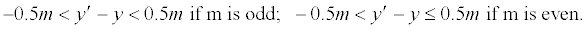(7)
Mean absolute error (mae) theorem (the formula to predict MAE of Stego): If the embedded data {x} is random, and the host has W×H elements; then after embedding in each element of the host a message digit by Eq. (1), the expected value for the Mean Absolute Error (MAE), which is defined asMAE = 0.25×m − 0.25/m if m is odd;
MAE=0.25×m if m is even. (8)
(Proof of MAE Theorem)
If m is odd, then 0.5m is not an integer; but 0.5(m−1) is. The Lemma states that −0.5m< y′−y <0.5m. From this and the case-bycase proof of the Lemma, we see that in a modulus-m system, the possible values of y′−y are the m consecutive integers {−0.5(m−1),…, −2, −1, 0, 1, 2, …, 0.5(m−1)} that satisfying the −0.5m< y′−y <0.5m requirement. The random distribution makes each integer here has similar occurrence rate (1/m). After taking absolute values and doing average for these m values, we get
[|−0.5(m−1)|+|−0.5(m−1)+1|…+|−2|+|−1|)+0+1+2+ …+(0.5(m−1)-1)+0.5(m−1)]/m
=2[1+2+ …+(0.5(m−1)-1)+0.5(m−1)]/m=[ 1 + 0.5(m-1)] (0.5(m-1))/m =( (0.5m)2 −0.25)/m=0.25m-0.25/m.
The case that m is even can be treated likewise. The m possible values of y′−y are {−(0.5m −1),…, −2, −1, 0, 1, 2, …, 0.5m −1, 0.5m }. After taking absolute values and then doing average, MAE= (0.5m +0 +2[1+2+3+…+(0.5m −1)] ) / m
= (0.5m +0+ (0.5m -1+1)( 0.5m −1) ) / m = 0.25m
-End of Proof for MAE Theorem-
Proof of Lemma: Let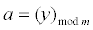then the original host value y satisfies
y=nm+a (9)
for some nonnegative integer n. Since both integers a and x are in the range {0,1,..,m-1}, then, as stated earlier, the possible values of a−x are
a−x ∈ {−m+1, -m+2, …, -2, -1, 0, 1, 2,, …, m-1}.
Therefore, −1< (a−x)/m <1 holds. Hence, round ((a−x)/m) ∈ { −1, 0, 1}.
Then,is either
(n−1)m+x, or nm+x, or (n+1)m+x. Together with Eq. (1) and (9),
we have(10)
Case 1
If 0.5m < (a−x) <m, then round ((ax)/m) = round (0.5…)=1. Eq. (10) implies that y′−y= −(a−x)+1m, which is a value in the range (− m+1m, −0.5m +1m), i.e. 0< y′−y <0.5m.
Case 2
If −0.5m < (a−x) <0.5m, then round ((a−x)/m) =0. Eq. (10) implies that y′−y = − (a−x), which is a value in the range −0.5m < y′−y <0.5m.
Case 3
If −m< (a−x) < −0.5m, then round ((a−x)/m) = −1. Eq. (10) implies that y′−y = − (a−x) −1m, which is a value in the range(0.5m−1m, m−1m), i.e. -0.5m< y′−y <0.
Case 4
If a=x±0.5m, which occurs only if m is an even number (because a and x are both integers), then y′−y = 0.5m, as proved below. There are two situations:
4a: if a=x+0.5m, then (1) and (9) give usTherefore, y′−y = 0.5m. The other situation is
4b: if a=x−0.5m, then Eq. (1) and (9) gives us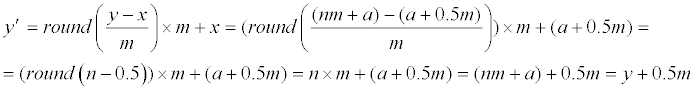Therefore, y′−y = 0.5m, again.
-End of the proof for the Lemma-

#### Results and Discussion

First we check whether the MAE formula Eq. (8) is good or not. We use four cover images {Lena, Baboon, F16 (Jet), Peppers}, each is 512-by-512, as host images. For each host image, we do ten experiments: five of the ten experiments use random data as the secret files, and the other five experiments use some natural images as the secret files. Table 3 shows the average of the ten experiments for each host image and for each m. We can see that the prediction formulas of MAE and PSNR are very useful because they are simple (e.g. MAE=0.25m if m is even; MAE=0.25m-0.25/m if m is odd) and very close to the experimental values. The PSNR values predicted by Property 3 , i.e. Eq. (3-4) here, is also very close to the experimental PSNR values. Notably, MAE=0.25m (or 0.25m-0.25/m if m is odd) means that the absolute error at each pixel is small. For example, when m=4, i.e. when bpp=log4/log2= 2, the MAE is MAE=0.25m=1; and hence, the absolute distortion |y′−y| at each pixel value y is 1 in average. Likewise, when m=8, i.e. bpp=log8/log2=3, the MAE is MAE=0.25×8=2; and hence, the absolute distortion |y′−y| at each pixel of gray value y is 2 in average. Note that the gray value y is in the range 0-255; so a distortion like 2 is not large.Table 3: MAE (Mean Absolute Error) and PSNR: predicted values vs. experimental results.
Below is a comparison of Methods [1,2] with some other methods reported recently. Since both Methods  and  can be expressed as Eq. (1-2) (except that Ref.  has a wider range of application because m is not necessarily in {2,4,8,16}), below we treat Methods  and  as a single method, i.e. the method using Eq. (1-2). Since this simple method emphasizes simplicity, high hiding capacity, and low distortion; we compare it with some other recently-published methods which also have high hiding capacity and low distortion.
Kanan and Nazeri  elegantly proposed a tuneable hiding method in spatial domain based on a genetic algorithm (GA). Their main idea is modeling the steganography problem as a searchingand- optimization problem. Then, in order to avoiding the exhausting searching of the optimized answer, a genetic algorithm is used in the searching of optimization. Their experimental results demonstrated that, in comparison with other popular steganography techniques, their algorithm not only achieves high embedding capacity but also enhances the PSNR of the stego images. According their Table 6, their PSNR values are about 45.63 dB when the hiding rate was 1.96 bits per pixel (bpp); whereas PSNR values of Thien and Lin  are about 46.37 dB at 2 bpp. Notably, larger bpp and larger PSNR are usually preferred, because larger larger bpp means higher capacity for hiding, and larger PSNR often means better stego image quality. Similarly, Kanan and Nazeri  has PSNR= 36.0 dB when bpp is 3.2; whereas Thien and Lin  has PSNR near 38.84 dB when bpp is 3.32. Finally, PSNR of Kanan and Nazeri  is near 35.01 dB when bpp is 3.95; whereas PSNR of Thien and Lin  is about 34.81 dB when bpp is 4 (or, about 35.42 dB when bpp is 3.91). Hence, the simpler and faster method (just using Eq. (1) and (2) for coding and decoding, resp.) of Thien and Lin  can compete with the Gene-Algorithm-searching optimization method introduced in study by Thien and Lin .
The data hiding method proposed by Tang et al. , is a gorgeous reversible method based on maximizing the difference values between neighbouring pixels. The conclusion of  stated that “….gains good PSNRs. compared to the scheme INP , the experimental results of proposed information hiding scheme improved the capacity. Therefore, this shows fully the better performance of the proposed scheme.” Now, if we compare the simple method (our Eq. (1-2)) here with the high-capacity method introduced in Ref.  which uses multilayer embedding; then, according to Tables 1 and 2 of Tang et al. , the scheme in  can offer an average payload 1.79 bpp and average PSNR value 33.85 dB, whereas the simple method (Eq. (1- 2)) here can offer average PSNR value 46.37 dB if 2 bpp (i.e. m=4 in Eq. (1-2)) is used. Again, the PSNR-bpp performance of the simple method using Eq. (1-2) is competitive. But, of course, their method has the reversible ability to recover the host image, and this is a feature that we do not have.
Below we compare the 2003 simple method (Eq. (1-2)) with a sophisticated modulus-based method  introduced by Maleki et al., in 2014. There are two versions in : adaptive and non-adaptive. According to their experiments for their non-adaptive version , their PSNR values are 51.12-51.18 dB for 1 bpp; 46.35-46.38 dB for 2 bpp; 40.65-40.75 dB for 3 bpp; 34.75-34.89 dB for 4 bpp. These values are very close to the {51.14 dB; 46.37 dB; 40.73 dB; 34.81 dB} values predicted by our Eq. (4) here, i.e. very close to the values predicted by Property 3 of , with m=2,4,8,16; respectively (or equivalently, with bpp=1,2,3,4, respectively).
As for the adaptive version of Maleki et al. , their bpp can be noninteger; and PSNR values for Maleki et al.  are as in Table 1. We can see that the simple method here (Eq. (1-2)) is still very competitive.
Besides Maleki et al., , the method of Sadashiv and Rao  is also an adaptive method because in  each smoother block hides lesser number of bits compared to edged block. Our PSNR-bpp performance also can compete with this adaptive method . For example, when the cover image is the Couple image, their Table 3 shows that they obtain
{(44.70dB at 1.95bpp); (36.96 dB at 2.80bpp); (36.85db at 3.20bpp)};
whereas our simple method that uses Eq. (1) for hiding can generate
{(46.3dB at 2 bpp); (39.8dB at 3.17bpp); (38.1dB at 3.46bpp)}.
As a remark, Mean Absolute Errors (MAE) are not shown in most of the published papers (including the above papers) which hide data in gray value images, so we only compare the PSNR values in the above paragraphs. However, the readers are welcome to use our MAE table here to compare with the MAE of their new methods. Also, to give the readers some ideas of the MAE, we compare our MAE with the MAE obtained by the Raja et al.,  or El-Emam . Since El- Emam  study is for color images, the image Lena has 512*512*3 bytes, so the bpp should be explained as number of hidden bits per pixel byte. Therefore, just like dealing with gray-level Lena, we are still talking about how many hidden bits there are in one host byte. From this table, we can see that the method of Eq. (1) is also competitive in terms of MAE (smaller MAE is better, larger bpp is better).
Finally, as a conclusion, from the above comparison with the methods introduced in [4-10], we can see that the capacity-distortion performance of the simple method [1,2] is still very competitive nowadays. Therefore, we can say that the method is simple in coding/ decoding (see Eq. (1-2)), with low-distortion; of high hiding capacity, and equipped with simple formulas to predict excellently both MAE and PSNR of the stego images in a very easy manner.

#### Acknowledgement

The author would like to thank the reviewers for the valuable suggestions. These suggestions are appreciatively acknowledged. This work is supported by the grant MOST103-2221-E-009-119-MY3.

## References## Explore SciTechnol

###### Citations : 453

Journal of Computer Engineering & Information Technology received 453 citations as per Google Scholar report

## Recommended Conferences

### Global Summit on Robotics and Artificial Intelligence

Prague, Czech Republic

Tokyo, Japan

London, UK